# C Input and Output

Input means to feed some data into the system through the input devices like keyboard.

Output means to get the result or output of the feeded data from the system or simply, it is the output shown on the output devices like monitor.

## The C Standard Files

C programming language treats all the devices as files. So devices such as the display are addressed in the same way as files and here the following three files are automatically opened when a program executes to provide access to the keyboard and screen.

Standard File File Pointer Device
Standard input stdin Keyboard
Standard output stdout Screen

The file Input and Output, means to access the file for reading and writing purpose. But this tutorial will only explain you how to read values from the input devices and then how to print the values on the output devices. To learn about C file input output, then refer C File I/O. Now let's discuss about the getchar() and the putchar() function.

## C getchar() and putchar()

As you can see, the function is self-explaining, the getchar() is used in getting the character input whereas the putchar() is used in printing the character output. Here if you enter more than one character then all the character will lost except the first character since getchar() and putchar() is used only to read character i.e., single character. To read complete character (string) entered by the user, you have to use gets() and puts() function discussed later. To learn more about the getchar() and the putchar() functions then refer, getchar() and putchar().

### C getchar() and putchar() Examples

Here are four example programs listed, to understand the functions getchar() and the putchar() completely.

#### Example 1

```#include<stdio.h>
#include<conio.h>
void main()
{
int i;
clrscr();

printf("Enter a value: ");
i = getchar();
printf("You entered: ");
putchar(i);

getch();
}```

Here is the output of this C program:#### Example 2

```#include<stdio.h>
#include<conio.h>
void main()
{
char c;
clrscr();

printf("Enter a character: ");
c = getchar();
printf("You entered: ");
putchar(c);

getch();
}```

Here is the output of above C program: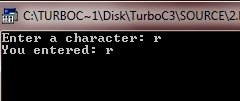#### Example 3

```#include<stdio.h>
#include<conio.h>
void main()
{
int num;
clrscr();

printf("Enter the number: ");
num = getchar();
printf("You entered: ");
putchar(num);

getch();
}```

Now enter more than one integer like 234 to check whether getchar() store this three digit integer or not. But as already told, it will not store, rather it will only store the first digit of the integer as shown here in this output: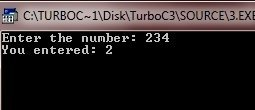#### Example 4

```#include<stdio.h>
#include<conio.h>
void main()
{
char str;
clrscr();

printf("Enter the string: ");
str = getchar();
printf("You entered: ");
putchar(str);

getch();
}```

Now enter more than one character (string) like "hello compiler" to check whether getchar() store all the character (or string) or not. But as already told it will not store, rather it will only store the first character of the string i.e., h as shown here in this output: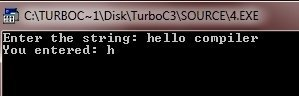Now let's discuss about the gets() and puts() functions.

## C gets() and puts()

The gets() function reads all the character entered by the user until a newline or EOF occur. In other word, the gets() function reads the string entered by the user. Whereas the puts() function writes the string to the output. To learn more about these gets() and the puts() functions in detail then refer, gets() and puts().

### C gets() and puts() Example

Here are the two example program to understand about gets() and puts() function completely.

#### Example 1

```#include<stdio.h>
#include<conio.h>
void main()
{
char str;
clrscr();

printf("Who are You ?\n");
gets(str);

printf("You are ");
puts(str);

getch();
}```

Here is the sample output of this C program: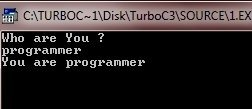#### Example 2

```#include<stdio.h>
#include<conio.h>
void main()
{
char line;
clrscr();

printf("Enter a line:\n");
gets(line);

printf("You entered:\n");
puts(line);

getch();
}```

Here is the sample output of the above C program: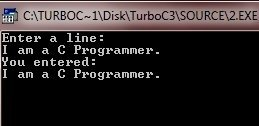Now let's discuss about scanf() and printf() functions.

## C scanf() and printf()

The scanf() function is used in reading the input from the standard input stream stdin and scans that input according to format provided. To learn more about C scanf() function, then refer scanf().

The printf() function is used in writing the output to the standard output stream stdout and produces the output according to a format provided. To learn more about C printf() function, then refer printf().

The format can be a simple constant string, but you can specify %s, %d, %c, %f, etc., to print or read strings, integer, character or float respectively. Now let's take some example programs.

### C scanf() and printf() Examples

Here are the two example programs listed to understand about the scanf() and the printf() functions completely.

#### Example 1

```#include<stdio.h>
#include<conio.h>
void main()
{
int i;
char str;
float f;
clrscr();

printf("Enter a value (integer): ");
scanf("%d", &i);
printf("You entered: %d", i);

printf("\n\nEnter the string: ");
scanf("%s", str);
printf("You entered: %s", str);

printf("\n\nEnter a value (float): ");
scanf("%f", &f);
printf("You entered: %f", f);

getch();
}```

Here is the sample run of this C program: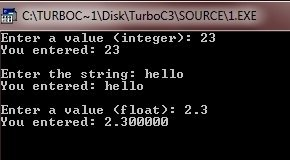#### Example 2

Now concentrate on this C program to understand the concept of scanf() function:

```#include<stdio.h>
#include<conio.h>
void main()
{
char str;
clrscr();

printf("Enter a string: ");
scanf("%s", str);
printf("You entered: %s", str);

getch();
}```

Now after running the above C program, just enter "hello compiler", you will see at the output screen, the " compiler" will lost. That is, you only see "hello". Because the function, scanf() stops reading as soon as it encounters a space so the string "hello compiler" have a space after 'o' of "hello", i.e, the scanf() only reads "hello" here and " compiler" will not be readed. So you will see only "hello" will produced as output by the function printf(). Here is the sample run of this C program.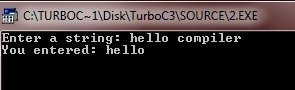Tools
Calculator